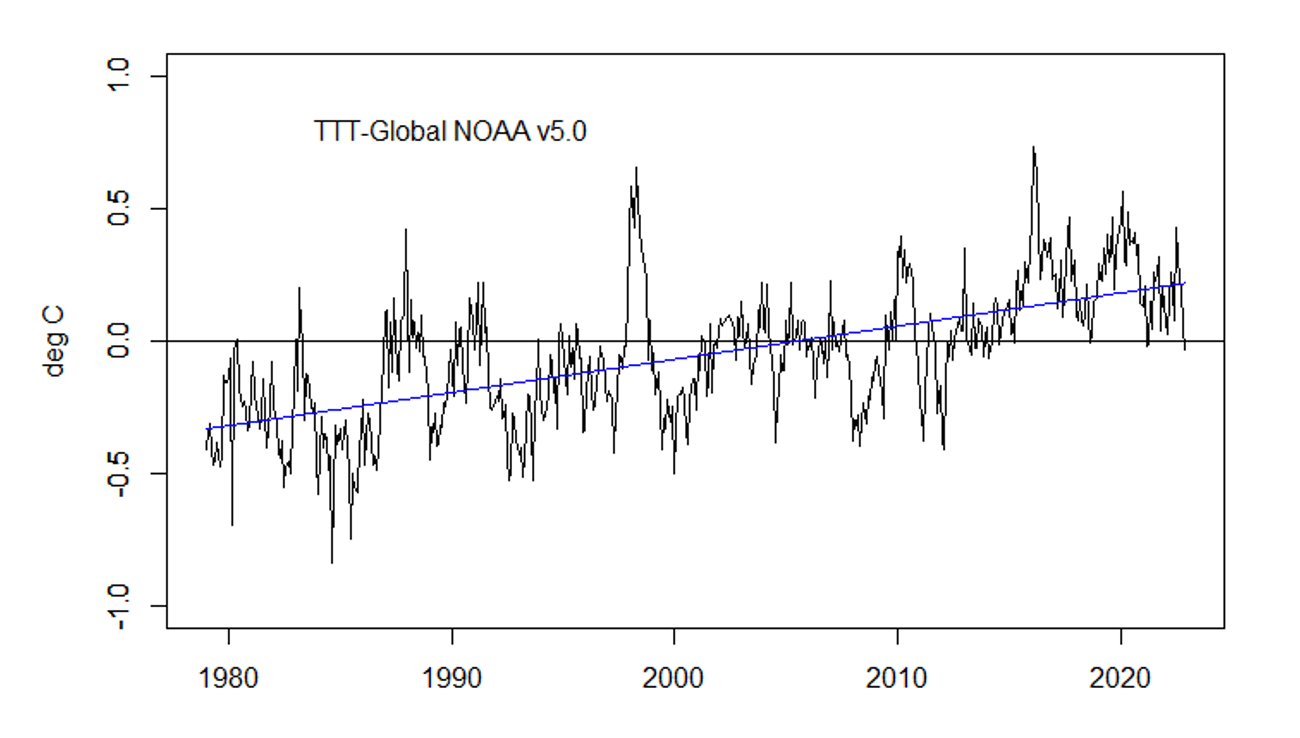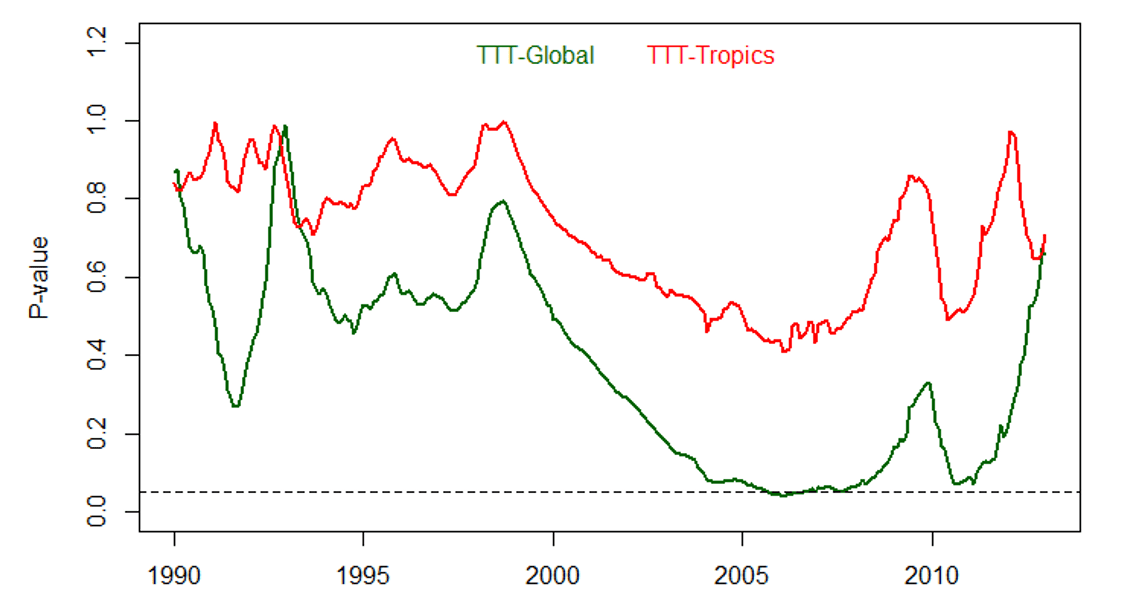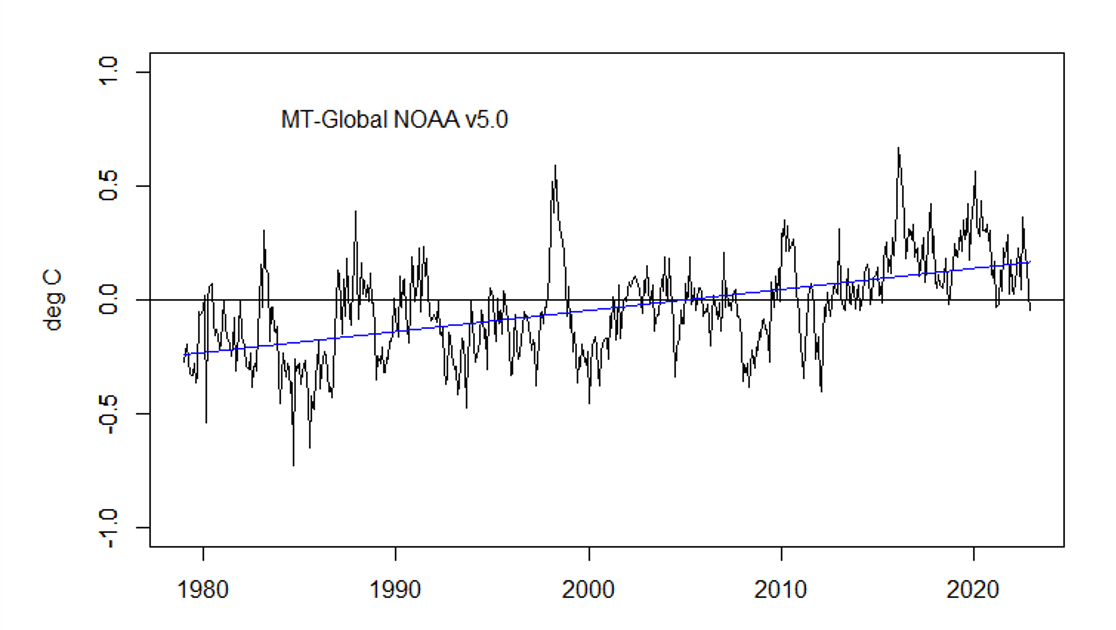# Is warming accelerating in the troposphere?

by Ross McKitrick

I  recently published an op-ed in the Financial Post describing the findings of the new JGR paper by NOAA’s Zou et al. NOAA’s STAR series of the MSU satellite-based tropospheric temperatures used to show more warming than UAH or RSS in the mid-troposphere. Zhou et al. recently rebuilt their dataset and now STAR has a slightly lower trend than UAH. This is a big deal because it adds to the evidence that GCMs are warming too much compared to observations, which suggests problems with their climate sensitivity (ECS) values.

In the ensuing discussion on Twitter and elsewhere, a few people criticized me for not drawing attention to Zou et al.’s observation that the warming rate appears to be accelerating, with the post-2001 trend about double that of the series as a whole. Apart from the limits of space in an op-ed, there are two reasons why I didn’t discuss that topic.

First, not too long ago many skeptics were pointing to the warming slowdown after 2001 as evidence that models were unreliable. The stock response on the part of modelers was to insist that we have to look at the whole data set to get the long-term picture right. Over short sub-intervals, so the argument goes, natural variability can cause departures above or below the trend, but this doesn’t take away from the reality of a long-term steady warming rate. Presumably the same logic still applies. It is especially the case when there was a pair of strong El Nino events after 2015 pushing the average tropospheric temperatures above trend. Of course, you will get an apparent acceleration if you compare a short sub-interval ending in 2020 with a trend using an earlier sample.

Second, if we want to test whether the warming rate has changed, it needs to be done properly. Zou et al. presented some suggestive calculations but not formal statistical tests. Suppose we have a data series Y(t) and we want to test if the trend changes after a chosen date which I’ll call g. The statistical question can be posed by using a regression model of the following form:

Y(t) = a0 + a1 x D(g) + a2 x T + a3 x D(g)T + e(t)

where a0 to a3 are regression coefficients, Y(t) is the temperature data series we are interested in, T is a time trend, and D(g) is a dummy variable, or binary indicator variable, taking the value 0 if the date is prior to the comparison point g and 1 if it is after; and e(t) are the error terms. For instance, Zou imposed a break point at 2001, so D(g) would be a vector consisting of zeroes up to December 2000 and ones thereafter. Note that D(g)T is a vector consisting of zeroes up to date g and the values of the time trend thereafter.

This form of the regression allows us to test if the trend changes after the date g. In the pre-g interval D(g) = 0 so the intercept is given by a0 and the trend is given by the coefficient a2. In the post-g interval D(g) = 1 so the intercept is given by the sum of a0 + a1 and the trend is given by the sum of a2+a3. Thus a1 measures if there was a step change and a3 measures if there was a change in trend between the pre- and post-g periods. To test if there was a significant change in the trend we use an F (or t) test of the restriction a3 =0. If the p-value on this test is above 0.05 we do not reject the null model and we can conclude there was no significant acceleration (or deceleration). Note that this allows for the alternative possibility that there was a step-change at g, but no change in the trend.

In order to do the test properly two further issues need to be addressed, only one of which I will deal with here. First, the error terms e(t) are autocorrelated, so an autocorrelation-consistent variance estimator is needed. It is common in climate applications to use an AR(1) model, but I will be using monthly data and this is likely inadequate. Instead I will use the Newey-West HAC method, which if you aren’t familiar with, think of it as being like AR(1) but more flexible so it is unbiased in large samples even if the autocorrelation process has more than just one lag.

[Digression: This further assumes that Y(t) is stationary around a deterministic trend. If this assumption is true then that raises large problems for attribution studies since GHG forcings are nonstationary and do not cointegrate. If that means nothing to you never mind. I will have much more to say on this at a later date.]

The second issue concerns how g is chosen. It is tempting to treat it as an unknown parameter and estimate it using an informal grid search. You can do that, but then you need to take account of the fact that you estimated it. It is common to experiment with values of g based on looking at the data series itself. Here is the total tropospheric temperature (TTT)-Global series from Zou et al.If you insert the break at various points to see what happens, this amounts to treating g as an unknown parameter to be estimated, but then inference regarding the other parameters is conditional on the value of g. I we perform the usual F test without taking account of the fact that g has been estimated, we will use incorrect critical values and overstate the significance of the test score. Tim Vogelsang and I discussed this issue in a 2014 paper in Environmetrics.

I’m going to ignore this issue for now but I’ll remark on it as we go along. I obtained from John Christy the new NOAA data showing the monthly TTT-Global and TTT-Tropics data (TTT= Total Tropospheric Temperature) from 1979:1 to 2022:12. I allowed g to vary month-by-month from 1990:1 to 2012:12, thus always allowing at least a decade before and after the break. For each placement of the break, I estimated the regression model described above and computed an F-test of the restriction a3 = 0 using the linear Hypothesis command in R with a Newey-West variance-covariance matrix.

The chart below shows the p-values of the test plotted over the assumed break date. The dotted line denotes the 5% significance level. Whenever the green or red line is below the dotted line we reject the hypothesis of no acceleration; in other words we have evidence of a significant change in the trend conditional on that break date.Looking at the global series (green), placing the break over most of the sample there is no evidence of acceleration but there is a brief interval in late-2005 through mid-2006 during which if you place the break there you can claim evidence of a significant acceleration in warming. But after that the tests go back into the non-rejection region, which means the apparent acceleration is temporary. Also we need to take account of the fact that we cherry-picked the date and since the p-value is so close to 0.05, any upward adjustment to the critical values will mean the rejection is no longer robust. Overall ,the TTT-global series does not support the claim of an accelerating trend. Further evidence is provided by the red line. If global warming in the troposphere was accelerating, then this presumably would also show up over the tropics. However, looking at the red line we see very clearly no evidence for acceleration regardless of the chosen break date.

The next figure shows the same results for the global LT (lower troposphere) and MT (mid troposphere) series. There is a bit more evidence of acceleration especially in the MT layer. But once again you have to cherry-pick the break date, and even then the tests move back into the non-rejection region if it is placed after mid-2011. Thus for most of the record we would conclude there is no robust evidence of a trend acceleration, even without adjusting the critical values for the fact that g is unknown.With regard to the mid-troposphere series we can say there is preliminary evidence of an acceleration, but the test scores reverse after 2010 so it is not yet a permanent feature of the data. Here is the time series of the global MT from NOAA v5.0. The linear trend is 0.092 degrees C per decade. Visually it looks like the record jumped in 2015 and has been returning to the trend ever since. It will take another 5 years or more of data to identify a permanent acceleration.In sum, based on a preliminary analysis the new NOAA data do not support a claim that warming in the troposphere has undergone a statistically-significant change in trend. The Global and Tropical TTT series show no support for the claim. The Global MT series appears to show support but only if the break data is placed in a specific interval in the early part of the last decade, and more recently the tests do not support acceleration. Finally, all of these results are biased towards finding evidence of a trend break due to the treatment of g. Robust critical values could be generated, which I might get to someday if no one else does it first.

via Climate Etc.

https://ift.tt/S7b6dpq

April 19, 2023 at 10:33AM